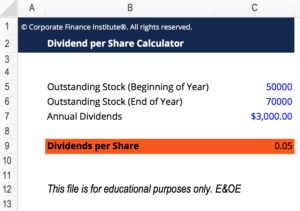# Dividend Per Share (DPS) Calculator

## Dividend Per Share (DPS) Calculator

This Dividend Per Share (DPS) Calculator template will help you calculate the total amount of dividend attributed to each individual share outstanding of a company.  Calculating the dividend per share allows an investor to determine the amount of cash he or she will receive on a per share basis.

Here is what the template looks like:### The formula for Dividend Per Share

The formula for calculating dividend per share has two variations:

or

### More Free Templates

For more resources, check out our business templates library to download numerous free Excel modeling, PowerPoint presentation and Word document templates.

• Excel Modeling Templates
• PowerPoint Presentation Templates
• Transaction Document Templates